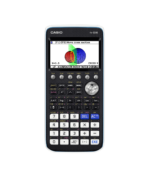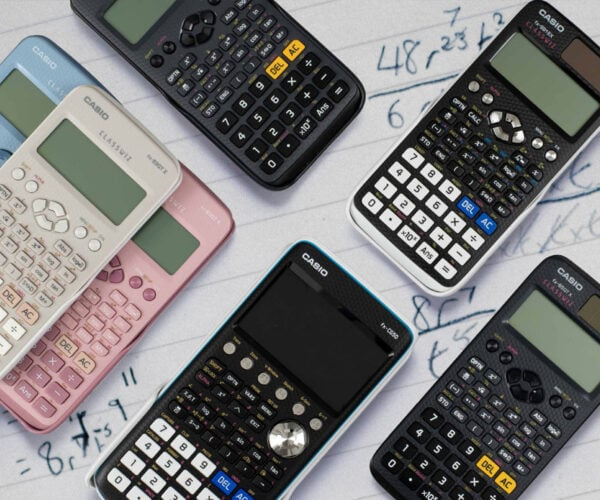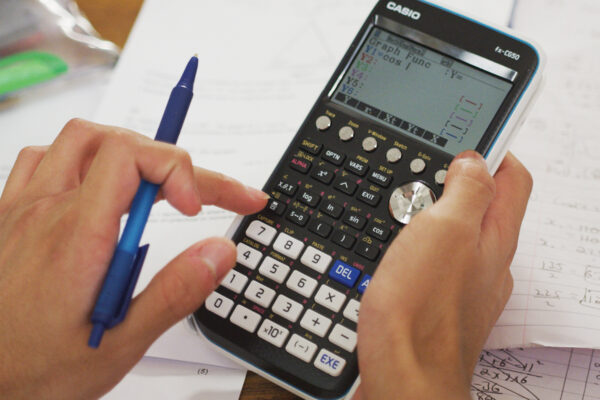A-Level - Casio Calculators

## A-Level## Students & Parents

At A-level you’ll be learning areas of maths you’ve not yet encountered. There will be new and more complex types of calculation. You will need at least an advanced scientific calculator to cover the minimum syllabus requirements.

Maths is a visual subject, and graphs form a significant part of A-level. A graphic calculator allows you to draw and analyse graphs. This will give you a significant advantage in lessons and exams. Our recommended graphic calculator is the fx-CG50.

## Scientific or graphic calculator?

There are two main types of calculator: scientific and graphic. A scientific calculator can only be used for calculation. A graphic calculator makes calculations, shows surds as exact answers and displays graphs. Maths is a visual subject, so the graphic calculator gives you a big advantage for learning and also in exams.

All Casio scientific and graphic calculators are allowed in exams.

## Related ResourcesReference
fx-CG50
fx-CG50 User Guide

Reference manual on how to operate every function on the fx-CG50 calculator. Cov…How to Leaflet
fx-CG50 A-Level GCSE IB
Run-Matrix Quick Start fx-CG50

How to enter basic calculations on the fx-CG50. Includes using ANS for simple it…How to Leaflet
fx-CG50 A-Level GCSE IB
Equation Quick Start fx-CG50

How to solve polynomial equations of order 2 and higher on the fx-CG50….How to Video
fx-CG50 A-Level GCSE IB
Simultaneous Equations – Solving Graphically

How to solve two simultaneous linear, or linear and quadratic, equations using g…How to Leaflet
fx-CG50 A-Level GCSE IB
Graph Quick Start fx-CG50

How to plot and change the view of a function on the fx-CG50. Includes tracing a…Reference Leaflet
fx-CG50

Overview of the menu functions of the fx-CG50, and the main control keys….### Calculator comparison

Casio offer a range of calculators for the large variety of maths, science and engineering courses across the United Kingdom and Ireland.### Learn how to Use the fx-CG50

Our extensive resources hub provides videos and pdfs, as well as ‘how to’ videos and downloads### Examinations

All calculator models sold by Casio UK are permitted in UK school exams. Some models are permitted in Irish and IB exams.## Should I Buy A Graphic Calculator?

Maths is a visual subject. A graphic calculator allows students to explore graphs. For example, you could plot a graph, find the coordinates where two graphs cross, or draw a data chart.

Graphic calculators make learning a lot easier and more fun. They also give an advantage in exams. We recommend the fx-CG50 calculator for more confident mathematicians, perhaps those taking the Higher tier. Our graphic calculators are allowed in GCSE exams. Explore the detailed specification of the calculator here.